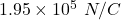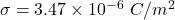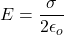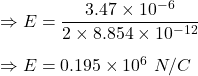## An infinitely large plane carries a uniformly distributed charge such that the charge per unit area is 3.47E-6 C/m2. What is the electric fi

Question

An infinitely large plane carries a uniformly distributed charge such that the charge per unit area is 3.47E-6 C/m2. What is the electric field magnitude |E| at a point that is 2.40 m from the plane

in progress 0
5 months 2021-08-05T21:14:12+00:00 1 Answers 4 views 0

## Answers ( )

1. Answer:Explanation:

Given

The charge per unit area on the plate isElectric for a infinitely large plane is given byTherefore, electric field at a point 2.4 m from the plane is